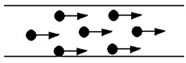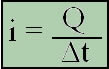Electricity & Megnetism

# Electricity

Electricity is a branch of physics that is divided into three parts: electrostatics, electrodynamics and electromagnetism.

1. Electrostatics: studies the effects produced by electrical charges at rest;
2. Electrodynamics: studies electrical charges in motion;
3. Electromagnetism: we see the effects produced by these charges in the space around this path.

In electricity, we learn that bodies are endowed with atoms that have protons, electrons, and neutrons, where protons are the positive subatomic particles and electrons, the negative particles.

Electric charges in orderly motion constitute an electric current. The charges that constitute this current are the free electrons:Electric current

The main factor of the electric current is to have the properties that the load at rest does not have, among such properties are:

• Thermal Effect – When the current passes through a conductor, producing heat and this conductor heats up.

• Magnetic Field – When current passes through a conductor and, around it, a magnetic field is produced, causing the current to behave like a magnet.

• Chemical Effect – The current produces an action called electrolysis.

• Physiological Effects –Pain and shock, caused by the fact that all living tissues have colloidal substances that, in turn, suffer the action of electricity. This action on the sensory nerves gives the sensation of pain, while on the motor nerves it gives the sensation of shock.

The intensity of the electric current is the division between the load and the variation of time, being represented by the following mathematical equation:Where:
i = current intensity (SI unit Ampere – A)
Q = electric charge (SI Coulomb unit – C)
Δt = time change (SI unit second – s)